# Efficient Query Filtering for Streaming Time Series Li

• Slides: 24
Download presentation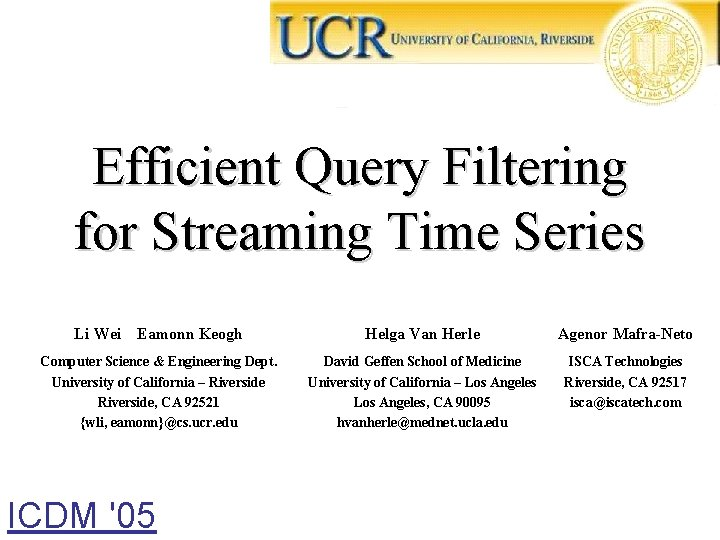Efficient Query Filtering for Streaming Time Series Li Wei Eamonn Keogh Helga Van Herle Agenor Mafra-Neto Computer Science & Engineering Dept. University of California – Riverside, CA 92521 {wli, eamonn}@cs. ucr. edu David Geffen School of Medicine University of California – Los Angeles, CA 90095 [email protected] ucla. edu ISCA Technologies Riverside, CA 92517 [email protected] com ICDM '05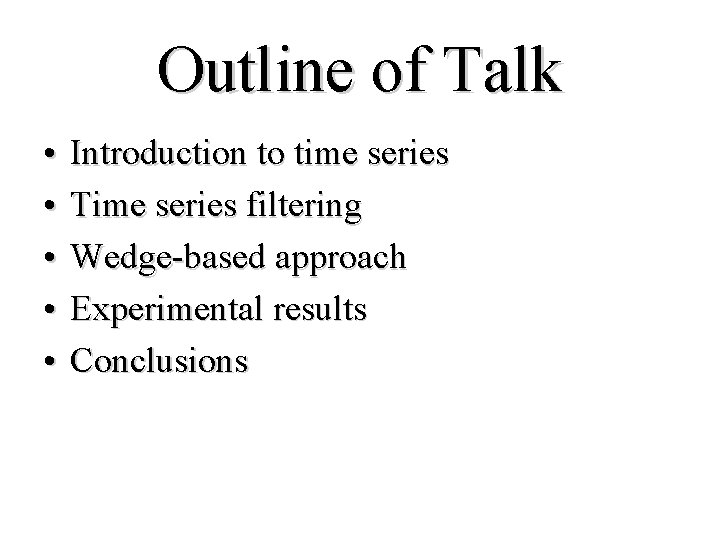Outline of Talk • • • Introduction to time series Time series filtering Wedge-based approach Experimental results ConclusionsWhat are Time Series? Time series are collections of observations made sequentially in time. 4. 7275 4. 7083 4. 6700 4. 6617 4. 6500 4. 6917 4. 7533 4. 8233 4. 8700 4. 8783 4. 8700 4. 8500 4. 8433 4. 8383 4. 8400 4. 8433. . .Time Series are Everywhere ECG Heartbeat Stock Image Video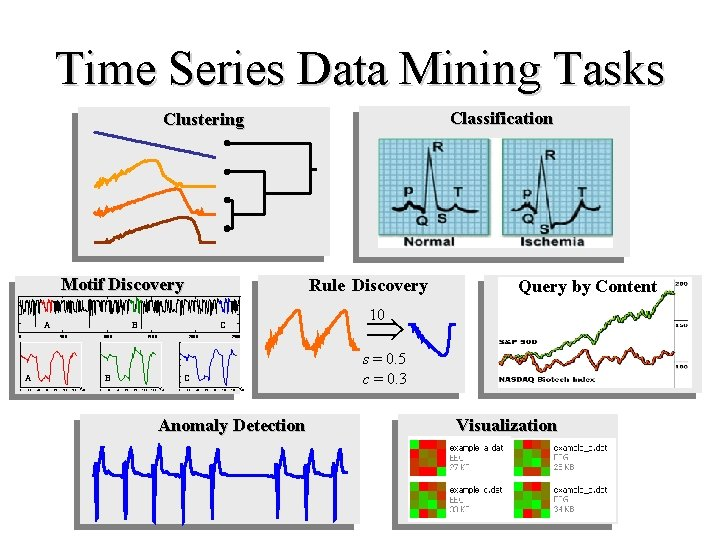Time Series Data Mining Tasks Classification Clustering Motif Discovery A B 0 50 0 1000 A 0 20 150 0 2000 60 80 100 120 140 0 20 2500 60 80 100 120 140 0 20 s = 0. 5 c = 0. 3 C 40 Query by Content 10 C B 40 Rule Discovery 40 60 80 100 120 140 Anomaly Detection Visualization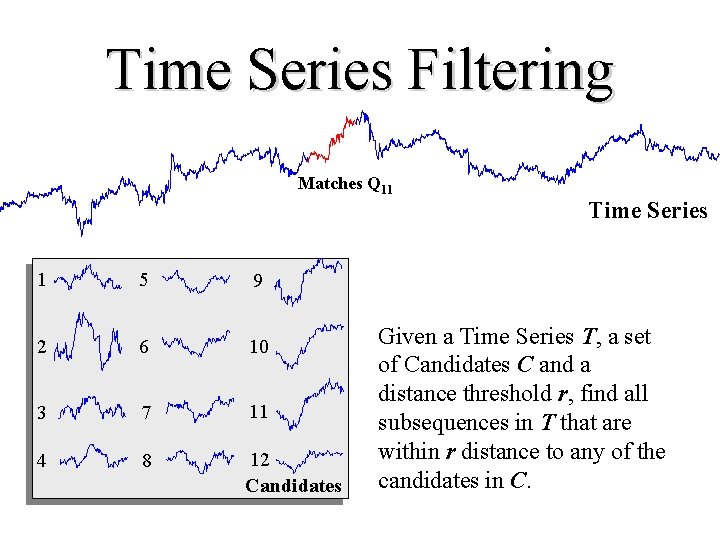Time Series Filtering Matches Q 11 Time Series 1 5 9 2 6 10 3 7 11 4 8 12 Candidates Given a Time Series T, a set of Candidates C and a distance threshold r, find all subsequences in T that are within r distance to any of the candidates in C.Filtering vs. Querying Query Database (template) Database Matches Q 11 Best match 1 5 9 1 6 2 6 10 2 7 3 8 4 9 5 10 3 7 11 4 8 12 Queries Database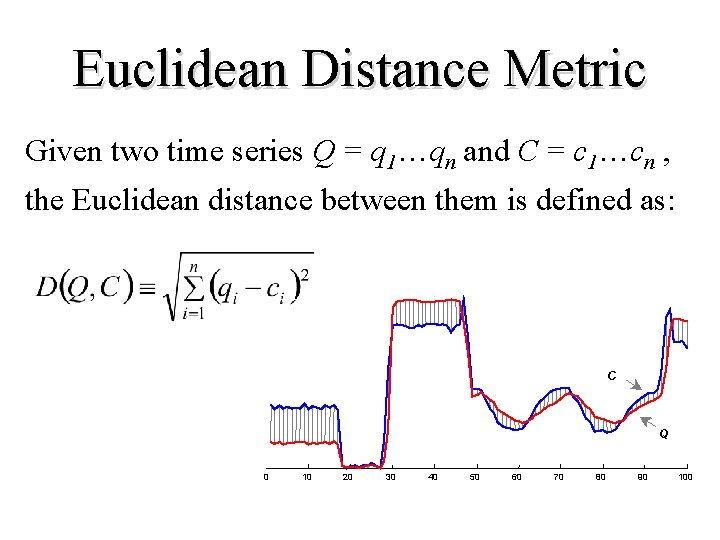Euclidean Distance Metric Given two time series Q = q 1…qn and C = c 1…cn , the Euclidean distance between them is defined as: C Q 0 10 20 30 40 50 60 70 80 90 100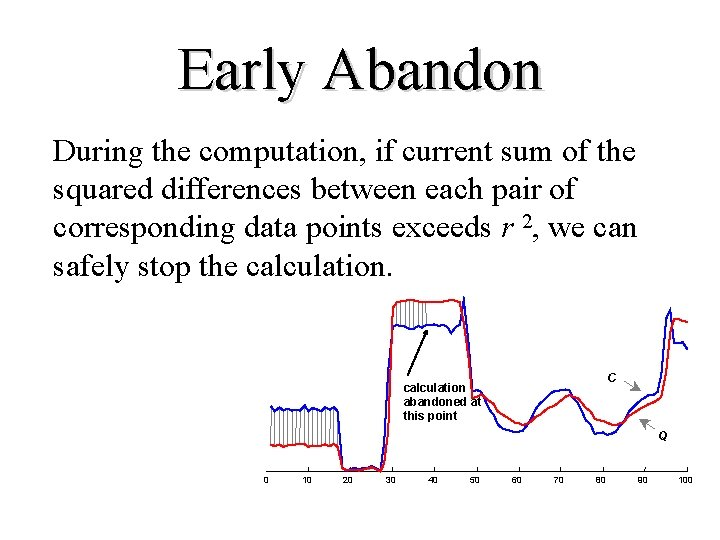Early Abandon During the computation, if current sum of the squared differences between each pair of corresponding data points exceeds r 2, we can safely stop the calculation. C calculation abandoned at this point Q 0 10 20 30 40 50 60 70 80 90 100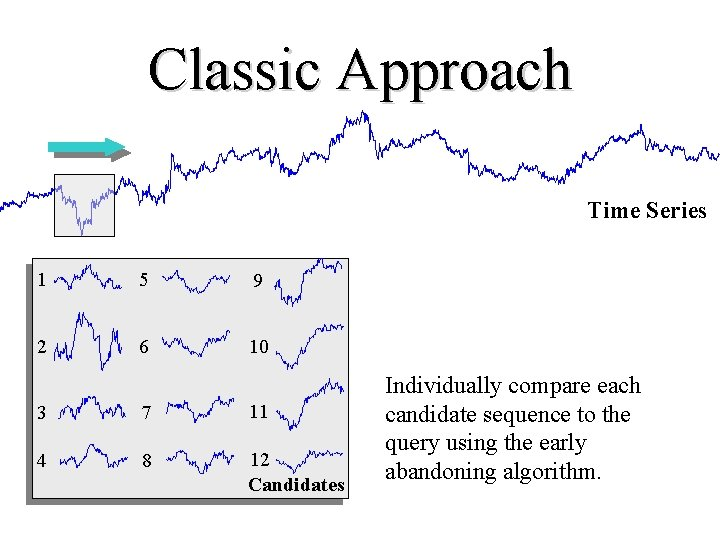Classic Approach Time Series 1 5 9 2 6 10 3 7 11 4 8 12 Candidates Individually compare each candidate sequence to the query using the early abandoning algorithm.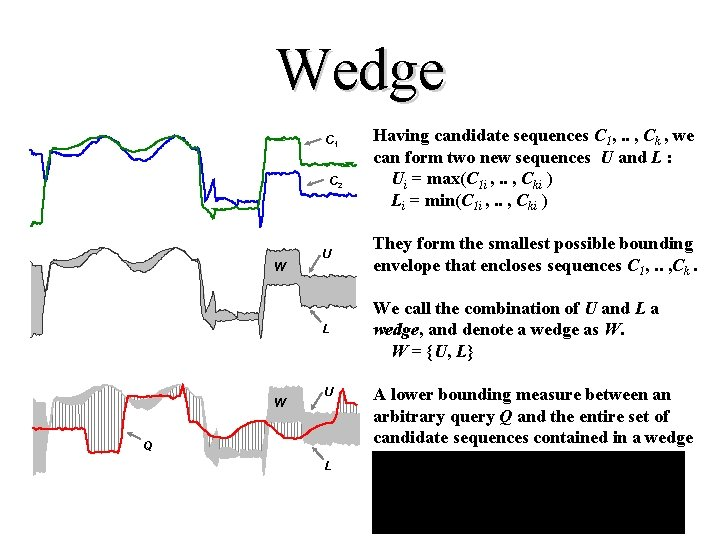Wedge C 1 C 2 W W Having candidate sequences C 1, . . , Ck , we can form two new sequences U and L : Ui = max(C 1 i , . . , Cki ) Li = min(C 1 i , . . , Cki ) U They form the smallest possible bounding envelope that encloses sequences C 1, . . , Ck. L We call the combination of U and L a wedge, and denote a wedge as W. W = {U, L} U Q L A lower bounding measure between an arbitrary query Q and the entire set of candidate sequences contained in a wedge W:Generalized Wedge • Use W(1, 2) to denote that a wedge is built from sequences C 1 and C 2. • Wedges can be hierarchally nested. For example, W((1, 2), 3) consists of W(1, 2) and C 3. C 1 (or W 1 ) C 2 (or W 2 ) W(1, 2) W((1, 2), 3) C 3 (or W 3 )Wedge Based Approach Time Series 1 5 9 2 6 10 3 7 11 4 8 12 Candidates • Compare the query to the wedge using LB_Keogh • If the LB_Keogh function early abandons, we are done • Otherwise individually compare each candidate sequences to the query using the early abandoning algorithm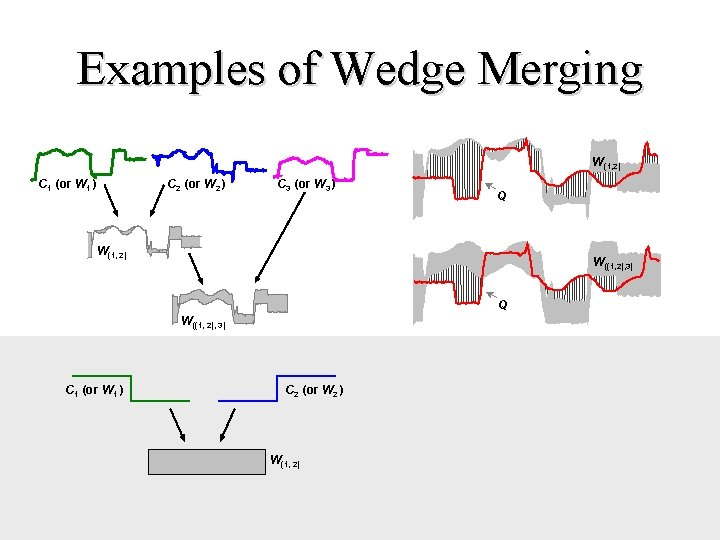Examples of Wedge Merging W(1, 2) C 1 (or W 1 ) C 2 (or W 2 ) C 3 (or W 3 ) Q W(1, 2) W((1, 2), 3) Q W((1, 2), 3) C 1 (or W 1 ) C 2 (or W 2 ) W(1, 2)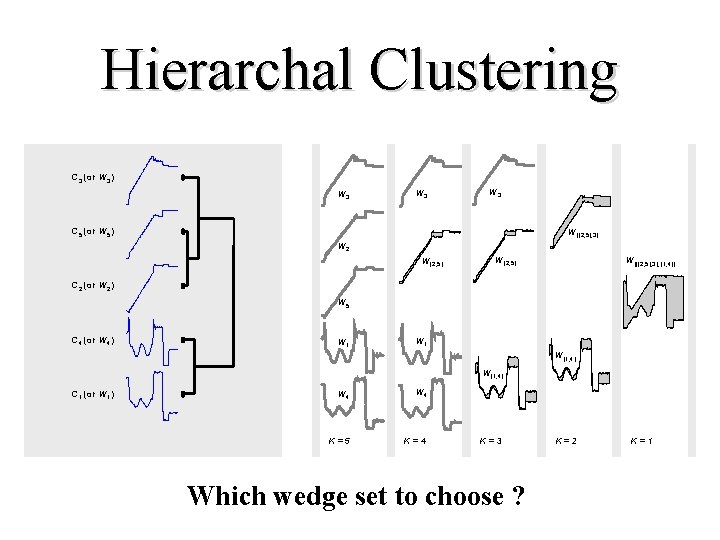Hierarchal Clustering C 3 (or W 3) W 3 W 3 C 5 (or W 5) W((2, 5), 3) W 2 W(2, 5) W(((2, 5), 3), (1, 4)) C 2 (or W 2) W 5 C 4 (or W 4) W 1 W(1, 4) C 1 (or W 1) W 4 K=5 K=4 K=3 Which wedge set to choose ? K=2 K=1Which Wedge Set to Choose ? • Test all k wedge sets on a representative sample of data • Choose the wedge set which performs the best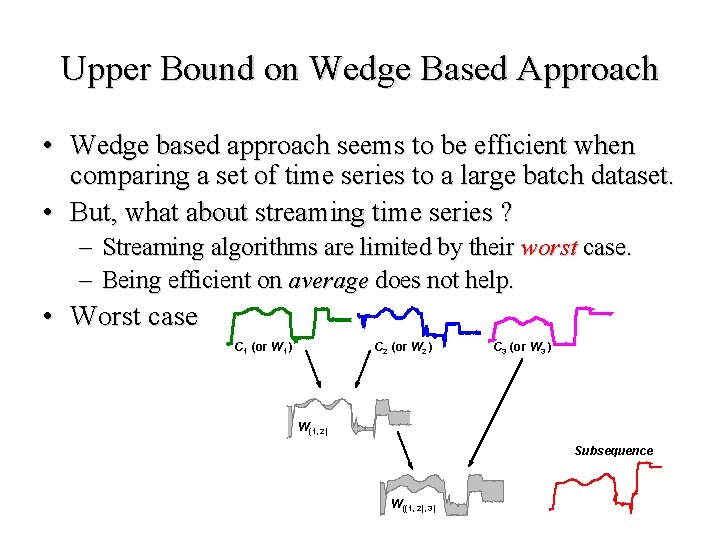Upper Bound on Wedge Based Approach • Wedge based approach seems to be efficient when comparing a set of time series to a large batch dataset. • But, what about streaming time series ? – Streaming algorithms are limited by their worst case. – Being efficient on average does not help. • Worst case C 1 (or W 1 ) C 2 (or W 2 ) C 3 (or W 3 ) W(1, 2) Subsequence W((1, 2), 3)If dist(W((2, 5), 3), W(1, 4)) >= 2 r Triangular Inequality Subsequence W 3 W 3 W((2, 5), 3) W 2 W(2, 5) W(((2, 5), 3), (1, 4)) <r W 5 W 1 W(1, 4) W 4 K=5 K=4 K=3 K=2 >= 2 r ? W 1 K=1 W(1, 4) cannot fail on both wedges fails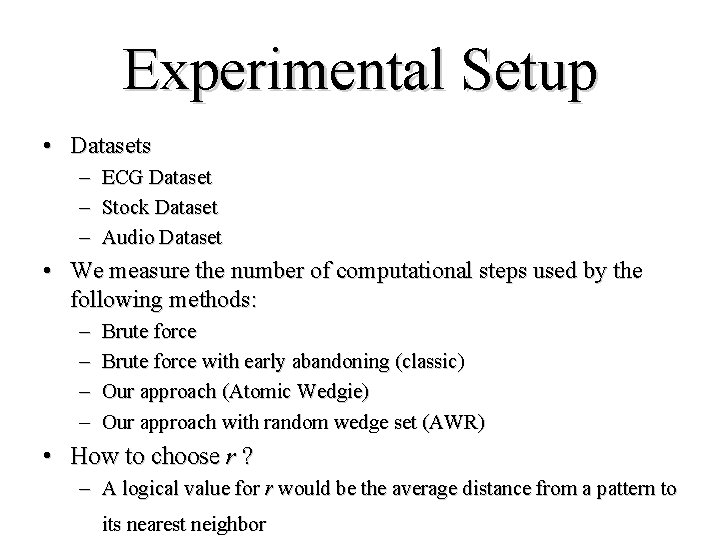Experimental Setup • Datasets – – – ECG Dataset Stock Dataset Audio Dataset • We measure the number of computational steps used by the following methods: – – Brute force with early abandoning (classic) Our approach (Atomic Wedgie) Our approach with random wedge set (AWR) • How to choose r ? – A logical value for r would be the average distance from a pattern to its nearest neighbor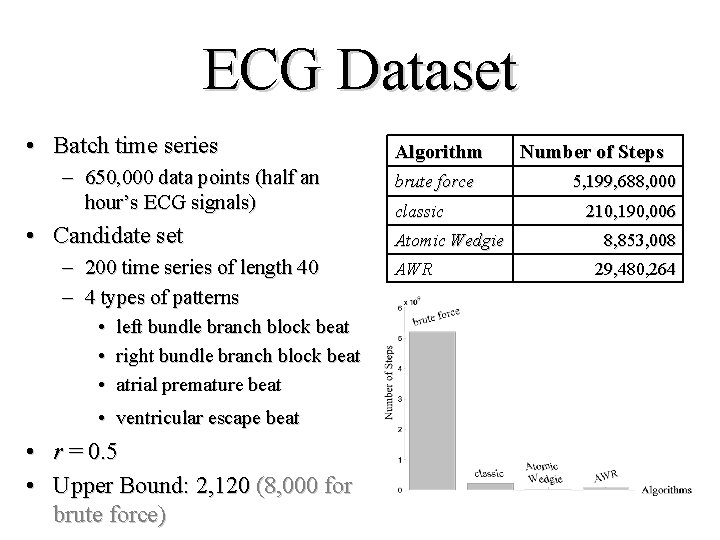ECG Dataset • Batch time series – 650, 000 data points (half an hour’s ECG signals) • Candidate set – 200 time series of length 40 – 4 types of patterns • left bundle branch block beat • right bundle branch block beat • atrial premature beat • ventricular escape beat • r = 0. 5 • Upper Bound: 2, 120 (8, 000 for brute force) Algorithm brute force classic Atomic Wedgie AWR Number of Steps 5, 199, 688, 000 210, 190, 006 8, 853, 008 29, 480, 264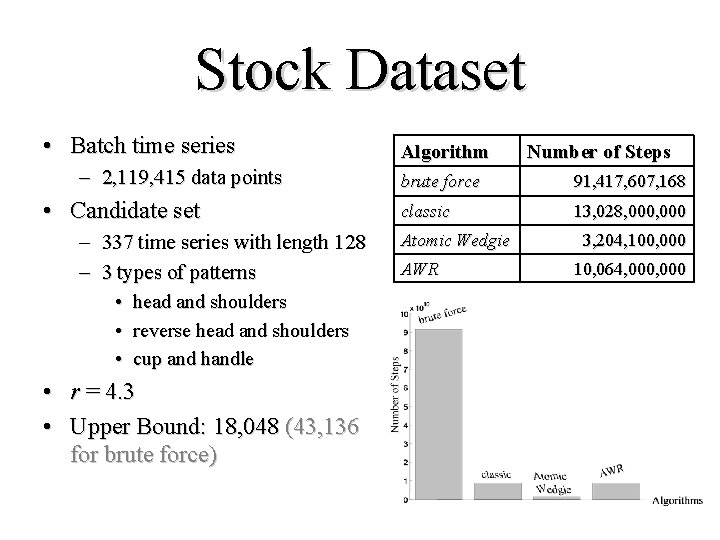Stock Dataset • Batch time series – 2, 119, 415 data points • Candidate set – 337 time series with length 128 – 3 types of patterns • head and shoulders • reverse head and shoulders • cup and handle • r = 4. 3 • Upper Bound: 18, 048 (43, 136 for brute force) Algorithm Number of Steps brute force 91, 417, 607, 168 classic 13, 028, 000 Atomic Wedgie AWR 3, 204, 100, 000 10, 064, 000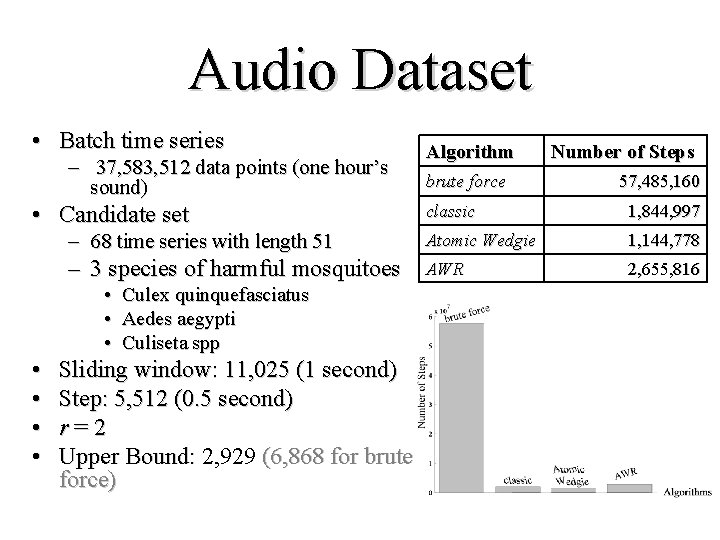Audio Dataset • Batch time series – 37, 583, 512 data points (one hour’s sound) • Candidate set brute force Number of Steps 57, 485, 160 classic 1, 844, 997 – 68 time series with length 51 Atomic Wedgie 1, 144, 778 – 3 species of harmful mosquitoes AWR 2, 655, 816 • Culex quinquefasciatus • Aedes aegypti • Culiseta spp • • Algorithm Sliding window: 11, 025 (1 second) Step: 5, 512 (0. 5 second) r=2 Upper Bound: 2, 929 (6, 868 for brute force)Conclusions • We introduce the problem of time series filtering. • Combining similar sequences into a wedge is a quite promising idea. • We have provided the upper bound of the cost of the algorithm to compute the fastest arrival rate we can guarantee to handle.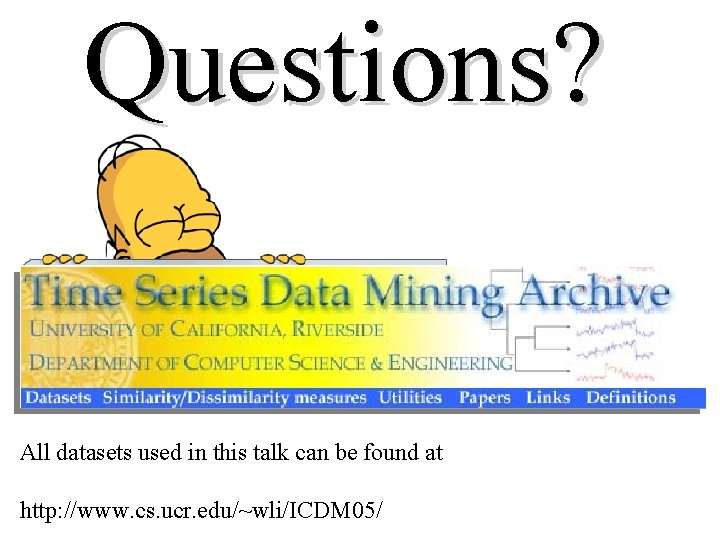Questions? All datasets used in this talk can be found at http: //www. cs. ucr. edu/~wli/ICDM 05/